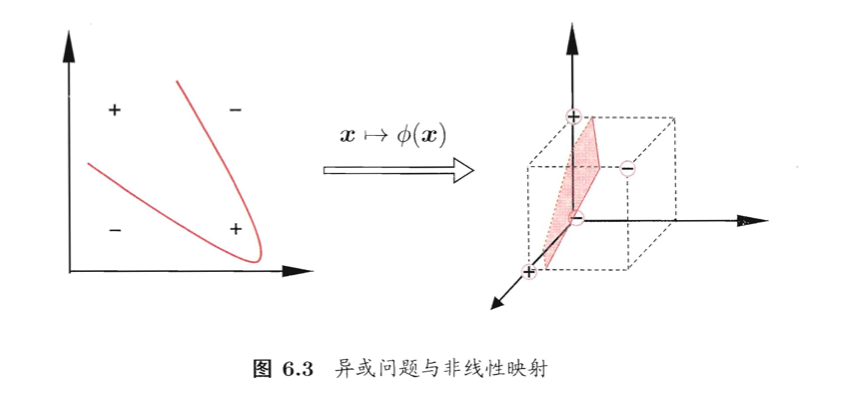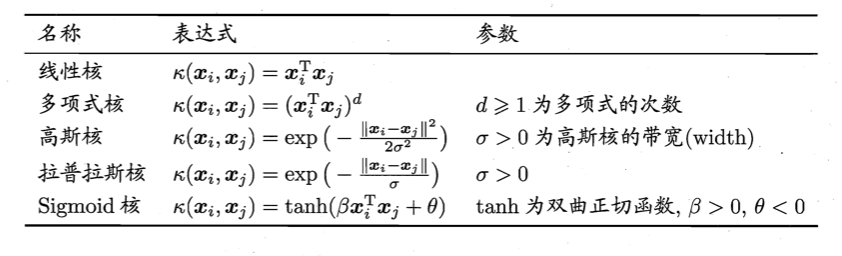# 【机器学习基础】第十八课：支持向量机之核函数

## 核函数，直积

Posted by x-jeff on January 3, 2021

【机器学习基础】系列博客为参考周志华老师的《机器学习》一书，自己所做的读书笔记。

# 1.核函数$f(\mathbf x)=\mathbf w ^T \phi (\mathbf x)+b \tag{1}$

\begin{align*} &\min \limits_{\mathbf w,b} \quad \frac{1}{2} \lVert \mathbf w \rVert ^2 \\ & \begin{array}{r@{\quad}r@{}l@{\quad}l} s.t.& y_i(\mathbf w^T \phi (\mathbf x_i) +b) \geqslant 1,i=1,2,...,m \\ \end{array} \end{align*} \tag{2}

\begin{align*} &\max \limits_{\mathbf \alpha} \quad \sum_{i=1}^m \alpha_i-\frac{1}{2} \sum_{i=1}^m \sum_{j=1}^m \alpha_i \alpha_j y_i y_j \phi(\mathbf x_i)^T \phi(\mathbf x_j) \\ & \begin{array}{r@{\quad}l@{}l@{\quad}l} s.t.& \sum_{i=1}^m \alpha_i y_i=0 \\ & \alpha_i \geqslant 0,i=1,2,...,m \\ \end{array} \end{align*} \tag{3}

$\kappa (\mathbf x_i,\mathbf x_j)=<\phi (\mathbf x_i)^T,\phi (\mathbf x_j)>=\phi (\mathbf x_i)^T \phi (\mathbf x_j) \tag{4}$

\begin{align*} &\max \limits_{\mathbf \alpha} \quad \sum_{i=1}^m \alpha_i-\frac{1}{2} \sum_{i=1}^m \sum_{j=1}^m \alpha_i \alpha_j y_i y_j \kappa (\mathbf x_i,\mathbf x_j) \\ & \begin{array}{r@{\quad}l@{}l@{\quad}l} s.t.& \sum_{i=1}^m \alpha_i y_i=0 \\ & \alpha_i \geqslant 0,i=1,2,...,m \\ \end{array} \end{align*} \tag{5}

\begin{align} f(\mathbf x) & = \mathbf w^T \phi(\mathbf x) +b \\ & = \sum_{i=1}^m \alpha_i y_i \phi (\mathbf x_i)^T \phi (\mathbf x) + b \\ & = \sum_{i=1}^m \alpha_i y_i \kappa (\mathbf x, \mathbf x_i) + b \end{align} \tag{6}

$\mathbf K = \begin{bmatrix} \kappa (\mathbf x_1,\mathbf x_1) & \cdots & \kappa (\mathbf x_1,\mathbf x_j) & \cdots & \kappa (\mathbf x_1,\mathbf x_m) \\ \vdots & \ddots & \vdots & \ddots & \vdots \\ \kappa (\mathbf x_i,\mathbf x_1) & \cdots & \kappa (\mathbf x_i,\mathbf x_j) & \cdots & \kappa (\mathbf x_i,\mathbf x_m) \\ \vdots & \ddots & \vdots & \ddots & \vdots \\ \kappa (\mathbf x_m,\mathbf x_1) & \cdots & \kappa (\mathbf x_m,\mathbf x_j) & \cdots & \kappa (\mathbf x_m,\mathbf x_m) \end{bmatrix}$

⚠️于是，“核函数选择”成为支持向量机的最大变数。若核函数选择不合适，则意味着将样本映射到了一个不合适的特征空间，很可能导致性能不佳。$d=1$时退化为线性核，高斯核亦称RBF核。

👉若$\kappa_1$和$\kappa_2$为核函数，则对于任意正数$\gamma_1,\gamma_2$，其线性组合

$\gamma_1 \kappa_1 + \gamma_2 \kappa_2$

👉若$\kappa_1$和$\kappa_2$为核函数，则核函数的直积

$\kappa_1 \otimes \kappa_2 (\mathbf x,\mathbf z)=\kappa_1(\mathbf x,\mathbf z) \kappa_2 (\mathbf x,\mathbf z)$

👉若$\kappa_1$为核函数，则对于任意函数$g(\mathbf x)$，

$\kappa (\mathbf x,\mathbf z)=g(\mathbf x)\kappa_1 (\mathbf x,\mathbf z)g(\mathbf z)$

# 2.直积

“直积”又称“笛卡尔乘积”：表示为$X \otimes Y$，第一个对象是$X$的成员而第二个对象是$Y$的所有可能有序对的其中一个成员。

$A \otimes B=\{(a, 0), (a, 1), (a, 2), (b, 0), (b, 1), (b, 2)\}$ $B \otimes A=\{(0, a), (0, b), (1, a), (1, b), (2, a), (2, b)\}$